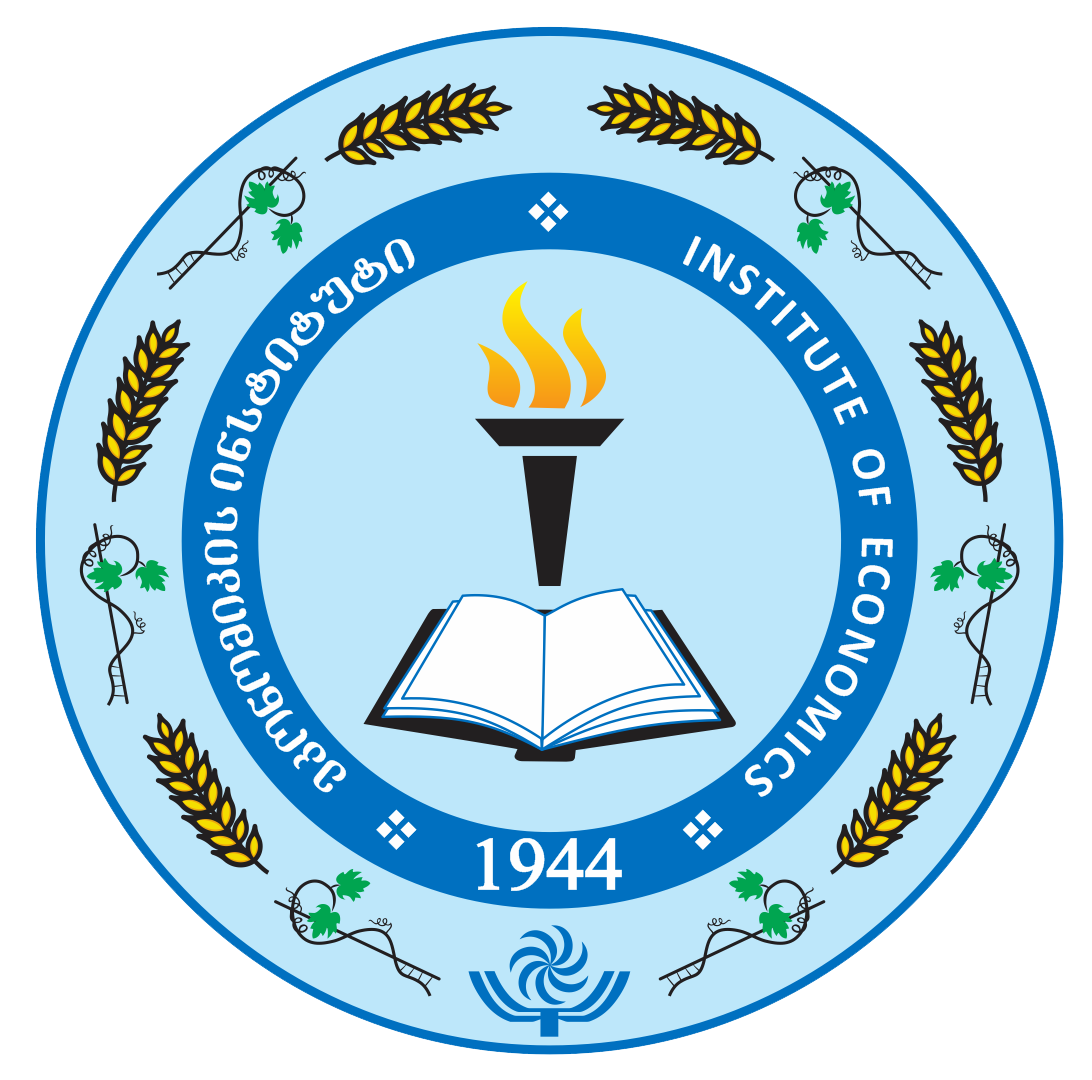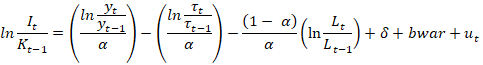EKONOMISTI   The international scientific and analytical, reviewed, printing and electronic journal of Paata Gugushvili Institute of Economics of Ivane Javakhishvili Tbilisi State UniversityHome ContactDOI: 10.36172/EKONOMISTI E      ISSN 2346-8432 Print ISSN 1987-6890 Journal number 4 ∘ Giorgi Miqeladze ∘ The multiplicative model of investment characterizing the deviations of the economy from the natural level and its empirical realization on the example of Georgian EconomySummapy. The article deals with the multiplicative model of investment characterizing the deviations of the economy from the natural level, adopted by consolidating the Cobb-Douglas theory,  theory of the natural level of economy and the theory of investment. In addition, on the example of Georgian economy, article empirically tests the efficiency of the model and its validity. The article describes the model of investment derived from the synthesis of Cobb-Douglas theory, the natural level of economy and investment theory:(1) By the multiplicative model of investment characterizing the deviations of the economy from the natural level is defined the link between the total number of investments, the amount of capital of the previous period, the GDP, the number of employees, the current and the lagging values ​​of the relative technological variable and the depreciation of capital. It is important to note that the results are based on several important assumptions, namely: 1. Permanent return to country's economy of scale; 2. Decreasing return to scale on capital and labor; 3. In every time period economy converges to its natural level; 4. Because of the changes in technology in each of the time period, the natural level of GDP is changing and it is defined as the product of the amount of natural level of the previous time period and the variables of the technological progress of the current and previous periods. In order to evaluate this model of investment on the example of the economy of Georgia the model (1) needs to involve a random member, a variable reflecting the results of the August 2008 war.(2) Furthermore, we do not have any empirical information about technological progress on the example of Georgia's economy and as it was noted in empirical realization of the investment acceleration model - on the principal capital depreciation rate [8, Mikeladze, p. 154-164]. If we assume that the depreciation rate of the principal capital is constant across time, than we can use two approaches for calculation its value and considering it in the model: 1. The value of the principal capital depreciation rate is calculated in assessing the investments’ acceleration model and we can consider it in evaluation of the model (2). 2. In the evaluation of the model (2) assess the capital depreciation rate as a free member in the model. Since the model does not contain a free member, it will not create a problem in the evaluation regarding what part it takes in the value of the evaluated coefficient. Before the evaluation of the model (2) we used the property of the logarithm concerning the difference of logarithms with one base and modified it as:(3) When evaluating the model (3), it is desirable to convert the dependent variable into logarithm as well, which in practical terms suggests that we will step to the relationship between variables in terms of growth rates:(4) Since we do not have information about the variable describing the technological progress, let’s check the model without this variable, this action will be reflected in the determinant coefficients. In order to determine the empirical values ​​of the dependent variable, namely for the values of the principal capital, we will use quarterly data ​​of the capital calculated by the investment acceleration model. By the empirical realization of the multiplicative model of investments characterizing the deviations of the economy from the natural level on the example of Georgian economy it is determined that the model is statistically suitable. The ration of gross investment and the lagged value of the principal capital are increasingly dependent on the ration of GDP's current and lagged values, and decreasingly dependent on the ration of current period employment with its lagged value. In addition, dependent variable is also decreasingly dependent on the war factor variable, which is a logical consequence. The value of the principal capital depreciation rate calculated using the investment acceleration model is a good estimate, since by the addition of an independent member in the model or by omitting it and re-evaluating the model we will get statistically insignificant results. Determination and corrected determination coefficients of the empirical model of investment indicate that the model lacks statistically significant other factor variable, which is a logical outcome. Before the empirical realization of the model it was noted that there was no information about the variable describing technological progress on the example of Georgia, so we examined the model without this variable. It could be said that this variable was reflected in the determination and corrected determination coefficients. These results indicate about the correctness of the theoretical model, which implies that the economy of the country converges to its natural level in every time period. The natural level changes in each of the time period through technological progress, which means that the upper limit of the country's economic capacity is changing.